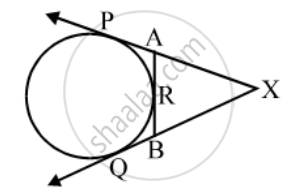# In fig. XP and XQ are tangents from X to the circle with centre O. R is a point on the circle. Prove that, XA + AR = XB + BR. - Mathematics

Sum

In fig. XP and XQ are tangents from X to the circle with centre O. R is a point on the circle. Prove that, XA + AR = XB + BR.

#### Solution

Since lengths of tangents from an exterior point to a circle are equal.∴ XP = XQ …. (i) [From X]

AP = AR …. (ii) [From A]

BQ = BR …. (iii) [From B]

Now, XP = XQ

⇒ XA + AP = XB + BQ

⇒ XA + AR = XB + BR [Using equations (i) and (ii)]

Concept: Concept of Circle - Centre, Radius, Diameter, Arc, Sector, Chord, Segment, Semicircle, Circumference, Interior and Exterior, Concentric Circles
Is there an error in this question or solution?
Share